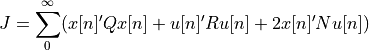# control.dlqr¶

control.dlqr(A, B, Q, R[, N])[source]

The dlqr() function computes the optimal state feedback controller u[n] = - K x[n] that minimizes the quadratic costThe function can be called with either 3, 4, or 5 arguments:

• dlqr(dsys, Q, R)

• dlqr(dsys, Q, R, N)

• dlqr(A, B, Q, R)

• dlqr(A, B, Q, R, N)

where dsys is a discrete-time StateSpace system, and A, B, Q, R, and N are 2d arrays of appropriate dimension (dsys.dt must not be 0.)

Parameters
• A (2D array) – Dynamics and input matrices

• B (2D array) – Dynamics and input matrices

• dsys (LTI StateSpace) – Discrete-time linear system

• Q (2D array) – State and input weight matrices

• R (2D array) – State and input weight matrices

• N (2D array, optional) – Cross weight matrix

• integral_action (ndarray, optional) – If this keyword is specified, the controller includes integral action in addition to state feedback. The value of the integral_action keyword should be an ndarray that will be multiplied by the current state to generate the error for the internal integrator states of the control law. The number of outputs that are to be integrated must match the number of additional rows and columns in the Q matrix.

• method (str, optional) – Set the method used for computing the result. Current methods are ‘slycot’ and ‘scipy’. If set to None (default), try ‘slycot’ first and then ‘scipy’.

Returns

• K (2D array (or matrix)) – State feedback gains

• S (2D array (or matrix)) – Solution to Riccati equation

• E (1D array) – Eigenvalues of the closed loop system

The return type for 2D arrays depends on the default class set for state space operations. See use_numpy_matrix().
>>> K, S, E = dlqr(dsys, Q, R, [N])